# Class 9A

On the final certificate have one quarter of the class 9A mark "C" of mathematics, seventh mark "C" from the Czech language and two students failed in chemistry. How many students attend class 9A?

n =  28

### Step-by-step explanation:Did you find an error or inaccuracy? Feel free to write us. Thank you!Tips to related online calculators
Do you want to calculate least common multiple two or more numbers?

## Related math problems and questions:

• 9.A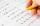9.A to attend more than 20 students but fewer than 40 students. A third of the pupils wrote a math test to mark 1, the sixth to mark 2, the ninth to mark 3. No one gets mark 4. How many students of class 9.A wrote a test to mark 5?
• Marks at schoolThere are 30 students in the class. Five students in the class had a mark three triple at the end of the math certificate, and the other students had a mark of one or two. The average mark in all students' mathematics in the class at the end of the year w
• Certificate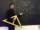There is 31 students in a class. From mathematics was'nt worse mark than 2. Average mark in mathematics was 2. How many students have mark 1 and how many mark 2?
• Three subjects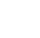In a class of 40 students, 18 passed mathematics, 19 passed accounts, 16 passed economics, 5 mathematics and accounts only, 6 mathematics only, 9 accounts only, 2 accounts and economics only, if each student offered at least one of the subjects. a) how ma
• Class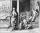When Pythagoras asked how many students attend the school, he said: "Half of the students studying mathematics, 1/4 music, seventh silent, and there are three girls at school." How many students had Pythagoras at school?
• Lcm = 22 + gcdThe least common multiple of two numbers is 22 more than their greatest common divisor. Find these numbers.
• Four classses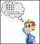Students of all 7, 8 and 9 classes in one school may take up 4,5,6 and 7 abreast and nobody will left. How many is the average count of pupils in one class if there are always four classes each grade?
• SchoolLess than 500 pupils attend school. When it is sorted into pairs, one pupil remains. Similarly, when sorted into 3, 4, 5 and 6 members team one remains. Sorted to seven members teams, no left behind. How many pupils are attending this school?
• LCMWhat is the least common multiple of 5, 50, 14?
• Ski class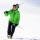Class attend 30 boys and some number of girls. Ski training was attended by 28 boys and all the girls, which was 95% of all students. How many girls attend this class? How many percent is that?
• Dentist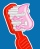Class 5. A participated in a preventive dental examination. A quarter of the pupils found two dental caries, an eighth of the pupils found one caries. Half the class had all their teeth healthy. How many students attend class 5. A if 4 students were missi
• Lcm simpleFind least common multiple of this two numbers: 140 175.
• Trams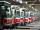Two trams started at the same time from the same place. One tram journey takes 30 minutes and the second 45 minutes to its final stop. How long will trams meet again?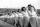Practitioners lined up in rectangle with row with four, five or six exercisers, one always missing to full rectangle. How many exercisers were on the field, if they have estimated not been more than 100?What is the Lcm of 120 15 and 5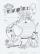The average of marks that have on the certificates students of 8A class in mathematics is exactly 2.45. If we did not add 1 and 3 of students Michael and Alena, who arrived a month ago, it would average exactly 2.5. Determine how many students have classA number is divisible by 24, 25, and 120 if it is increased by 20. Find the number.